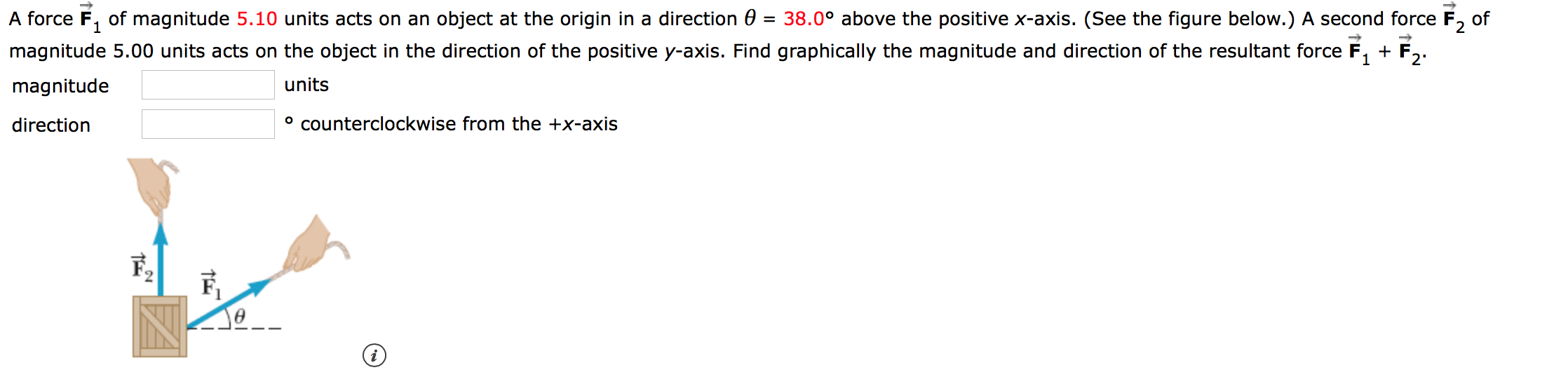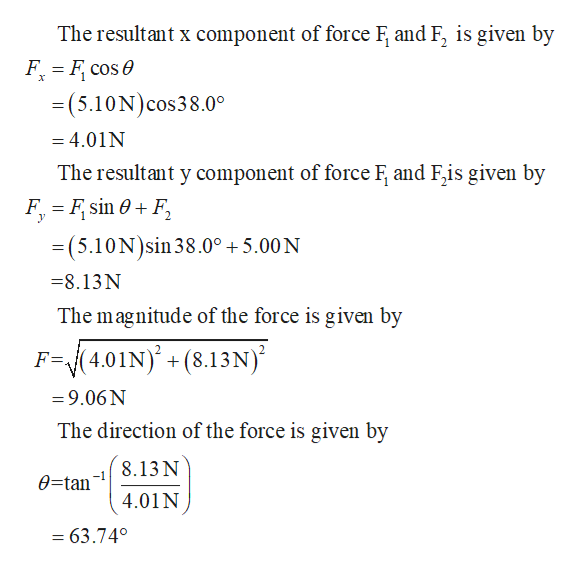# A force F of magnitude 5.10 units acts on an object at the origin in a direction 0 = 38.0° above the positive x-axis. (See the figure below.) A second force F2 ofmagnitude 5.00 units acts on the object in the direction of the positive y-axis. Find graphically the magnitude and direction of the resultant forceF12unitsmagnitudeocounterclockwise from the +x-axisdirection

Questionhelp_outlineImage TranscriptioncloseA force F of magnitude 5.10 units acts on an object at the origin in a direction 0 = 38.0° above the positive x-axis. (See the figure below.) A second force F2 of magnitude 5.00 units acts on the object in the direction of the positive y-axis. Find graphically the magnitude and direction of the resultant force F 1 2 units magnitude ocounterclockwise from the +x-axis direction fullscreen
check_circle

Step 1

The resultant force of F1 and F2 is resolved into F1cosθ along the horizontal direction , and ...help_outlineImage TranscriptioncloseThe resultant x component of force F and F, is given by F F cose =(5.10N) cos38.0° =4.01N The resultant y component of force F and F,is given by F F sin 0F = (5.10N)sin 38.0° 5.00 N =8.13N The magnitude of the force is given by (4.01N) + (8.13N) F=A =9.06N The direction of the force is given by 8.13 N 0=tan 4.01 N = 63.740 fullscreen

### Want to see the full answer?

See Solution

#### Want to see this answer and more?

Solutions are written by subject experts who are available 24/7. Questions are typically answered within 1 hour.*

See Solution
*Response times may vary by subject and question.
Tagged in

### Newtons Laws of Motion